# 3.2    2D and 3D Delaunay Triangulation

The Delaunay triangulation and the Voronoi diagram are dual structures and contain the same information in different form. Computing one of these structures does automatically create the other. Furthermore there is a connection between Delaunay triangulations and convex hulls in one higher dimension. The Delaunay triangulation and the Voronoi diagram in d dimensions can be constructed from a convex hull in d+1 dimensions. The Delaunay triangulation for a set of d-dimensional points is the projection of the points of the hull in d+1 dimensions. A introduction can be found in [ ORo98 ].

• 2D Delaunay Triangulation

Two algorithmic variants of the 2D Delaunay triangulation have been realized. One is the incremental algorithm [ Lis94 ] and the other the recursive variant with a ''divide and conquer'' strategy [ GSt85 ]. The two following images show the Delaunay triangulation (Figure 4a) and the corresponding Voronoi diagram (Figure 4b), created from 5964 points.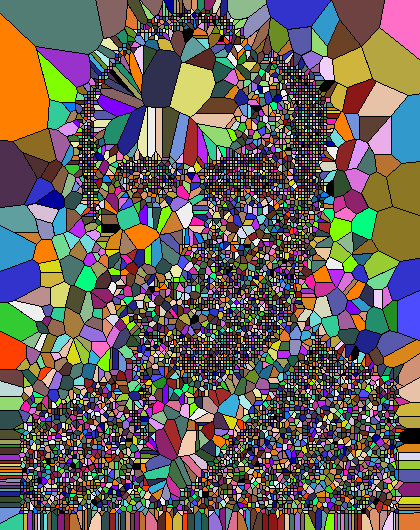(a) Delaunay triangulation (b) Voronoi diagram
Figure 4: Delaunay triangulation or Voronoi diagram in 2D

Figure 5 shows both, the Delaunay triangulation and the Voronoi diagram, in one image, for a set of 10 points.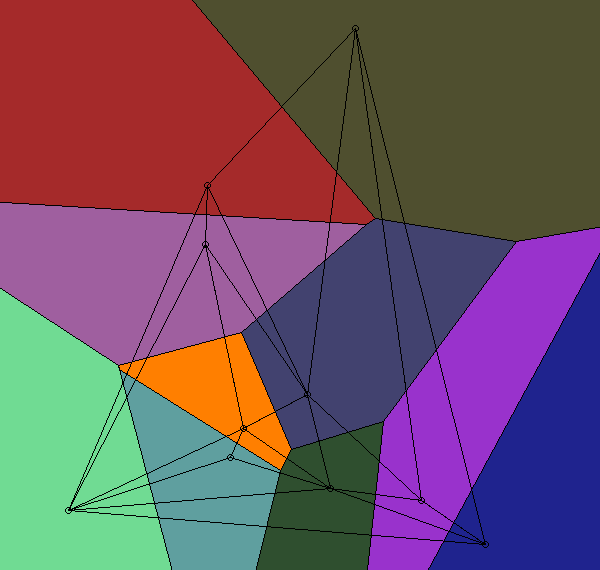Figure 5: Delaunay triangulation and Voronoi diagram in 2D

• Voronoi Diagram on the Sphere

Figure 6 shows a Voronoi diagram, created from the positions of the capitals of 228 countries (including overseas territories and the like) blended with the world map. The red dots show the GPS positions of the capitals, which have been transformed to 3D points using the WGS-84 [ WGS84 ] ellipsoid. The diagram has been created from the convex hull of these points. The following PDF document contains a 3D model with this Voronoi diagram: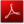. Figure 7 shows this Voronoi diagram of the whole world in 2D.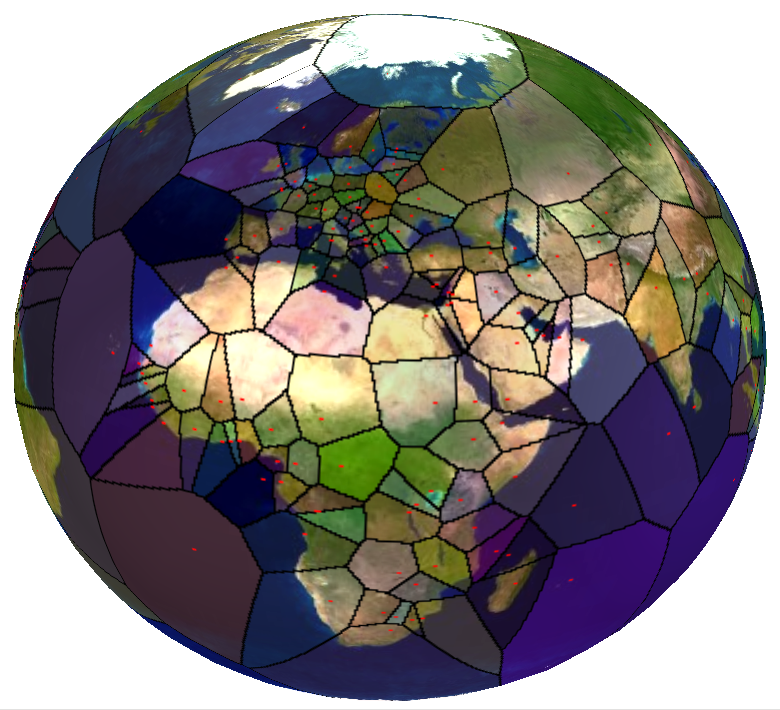Figure 6: Voronoi diagram on the sphere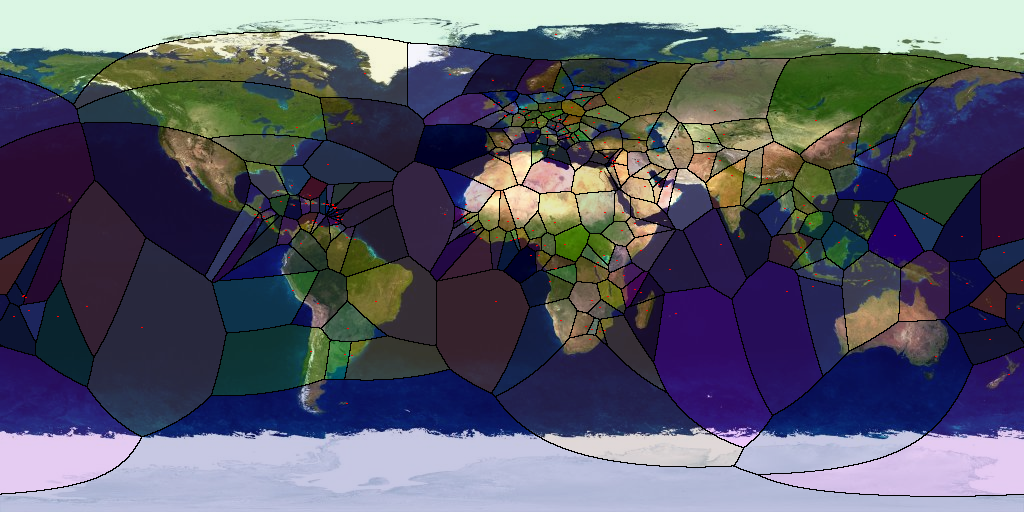Figure 7: Voronoi diagram of the whole world

• 3D Delaunay Triangulation

The implementation of the 3D Delaunay triangulation is based on the algorithm described in [ ESh96 ]. An extensive description (in German) can be found in [ BKl06 ].

The following two images (Figure 8a and Figure 8b) show the well known Stanford Bunny. The right one shows a 3D Delaunay triangulation of 71 weighted points of its point set.(a) Stanford Bunny (b) 3D Delaunay triangulation of the bunny
Figure 8: Bunny 3D Delaunay Triangulation

The 3D Delaunay triangulation for point sets with symmetries can be generated with the help of Simulation of Simplicity [ EMu90 ]. The following two images (Figure 9a and Figure 9b) show an icosahedron. The right Figure 9b shows a possible 3D Delaunay triangulation of this platonic solid.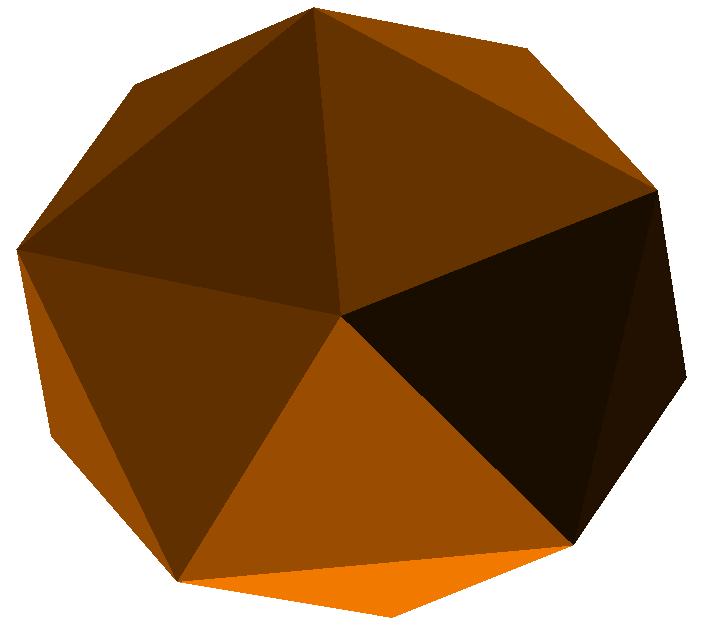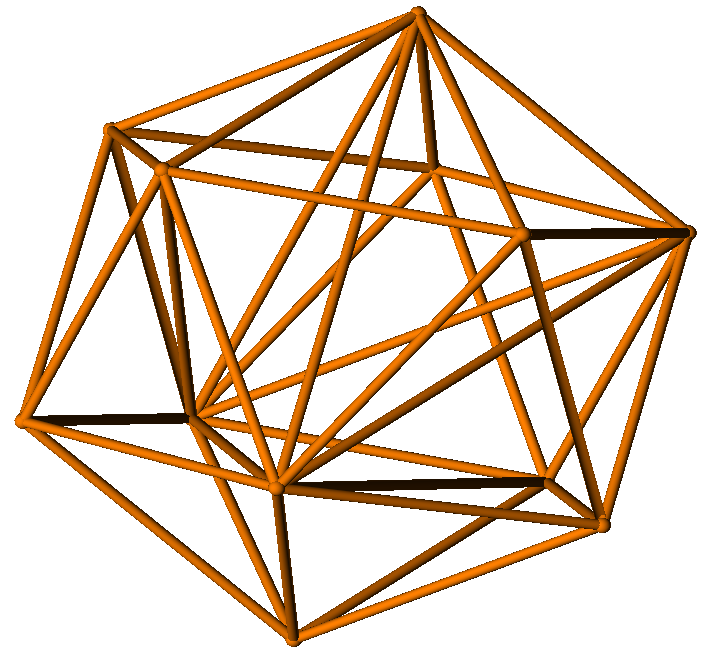(a) Icosahedron (b) 3D Delaunay triangulation of an icosahedron
Figure 9: 3D Delaunay Triangulation of Icosahedron

The following PDF document contains 3D models of 3D Delaunay triangulations:.